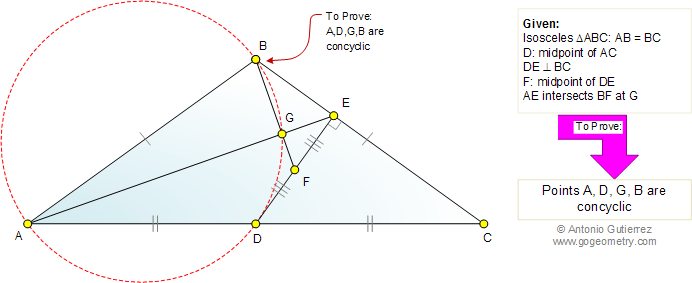# Geometry Problem 577: Isosceles triangle, Midpoint, Perpendicular, Concyclic points, Circle. Level: Math Education, High School, College

 The figure shows an isosceles triangle ABC (AB = BC). D is the midpoint of AC and DE is perpendicular to BC. F is the midpoint of DE and AE intersects BF at G. Prove that the points A, D, G, and B are concyclic (lie on a same circle).Home | Search | Geometry | Problems | All Problems | Visual Index | 571-580 | Email | Post a comment or solution | By Antonio Gutierrez